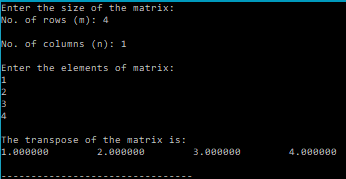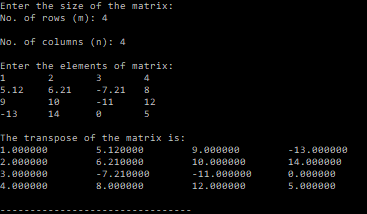# Transpose of a Matrix – C PROGRAM

The transpose of a matrix is the matrix obtained by replacing all elements$a_{ij}$ with$a_{ji}$

The following C code let’s the user enter a matrix,$A_{m\times n}$ and returns it’s transpose,$A^T_{n\times m}$.

### CODE:

/**************************************************
*************MATRIX TRANSPOSE*****************
**************************************************/
#include<stdio.h>
/*******
Function that calculates the transpose of matrices:
There are two options to do this in C.
1. Pass a matrix (trans) as the parameter, and calculate and store the transpose in it.
2. Use malloc and make the function of pointer type and return the pointer.
This program uses the first option.
********/
void matTranspose(int m, int n, double a[m][n], double trans[n][m]){
int i,j,k;
for(i=0;i<n;i++){
for(j=0;j<m;j++){
trans[i][j]=a[j][i];
}
}
}
/*******
Function that reads the elements of a matrix row-wise
Parameters: rows(m),columns(n),matrix[m][n]
*******/
void readMatrix(int m, int n, double matrix[m][n]){
int i,j;
for(i=0;i<m;i++){
for(j=0;j<n;j++){
scanf("%lf",&matrix[i][j]);
}
}
}
/*******
Function that prints the elements of a matrix row-wise
Parameters: rows(m),columns(n),matrix[m][n]
*******/
void printMatrix(int m, int n, double matrix[m][n]){
int i,j;
for(i=0;i<m;i++){
for(j=0;j<n;j++){
printf("%lf\t",matrix[i][j]);
}
printf("\n");
}
}
int main(){
int m,n,i,j;
printf("Enter the size of the matrix:\nNo. of rows (m): ");
scanf("%d",&m);
printf("\nNo. of columns (n): ");
scanf("%d",&n);
double a[m][n];
double trans[n][m];
printf("\nEnter the elements of matrix:\n");
matTranspose(m,n,a,trans);
printf("\nThe transpose of the matrix is:\n");
printMatrix(n,m,trans);
}


### OUTPUT:### Android Apps:

I’ve also created a few Android apps that perform various matrix operations and can come in handy to those taking a course on Numerical Methods.